# Maths problem help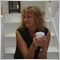264

Feeling stupid because I just cannot work it out, and wonder if anyone here can give me a hand?

I want to work out the following for a spreadsheet calculation

If the average of my shorts are   8 lots at 12067 price level

And the average of my longs are  5 lots at 11979 price level

What level is my break even?

*Calculation formula needed, as I have a lovely indicator that gives me this figure in realtime

on my charts, but I want to put the calculation on my spreadsheet for a projectory basis.

Just can't work it out, so any help very much appreciated.

Regards

Kate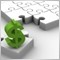11092

kate682:

Feeling stupid because I just cannot work it out, and wonder if anyone here can give me a hand?

Hiya Kate

OK, I'll give it a go! ;)

Assuming your spread sheet looks like this

AB
1Sell LotsSell Price
2Buy LotsBuy Price

So in your example:

AB
1812067
2511979

Your calculation cell would be:

`=B1-((B1-B2)/(A1+A2))*A2`

So in your example, the breakeven would be 12033.15

I haven't checked it thoroughly so let me know if that doesn't work and I'll give it another shot :D

Cheers
Stu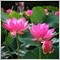53874

Filter:

So in your example, the breakeven would be 12033.15

It is wrong. at this price, both buy and sell have profit.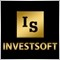39908

Check this method:

A (Lots)B (Price)C (Lots*Price)
1-812067-96536
251197959895

BE = SUM(C1:C2)/SUM(A1:A2)

BE = 12213,67

If number of lots for BUY and for SELL is equal than BE level doesn't exist.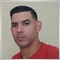847

Base on my calculations this is what I got 12012

12067-11979= 88

88x5= 440

88x8 = 704

704-440=264

8x33=264

88-33=55

55x8=440

12067 -55 =12012

So there you got the breakeven number

12012

Please be advise you said break even you didn't mention spread on pair53874

forex_trader:

BE = 12213,67

it is ok if have no spread and swap.11092

tuoitrecuoi:
It is wrong. at this price, both buy and sell have profit.

Ahh yes, that's what I get for trying maths problems too late at night :)

My equation is actually still correct, but the sell lots should have been negated (ie -8, not +8). You then get the BE of 12213.67. And as FT said, if lots are equal there is no BE and both his equation and mine will fail with a DIV 0 error. So you would need to also put a check in for that.

So if you have negative sell lots:

```=IF(A1+A2=0,"No Breakeven Possible",B1-((B1-B2)/(A1+A2))*A2)

```
Great team work :)